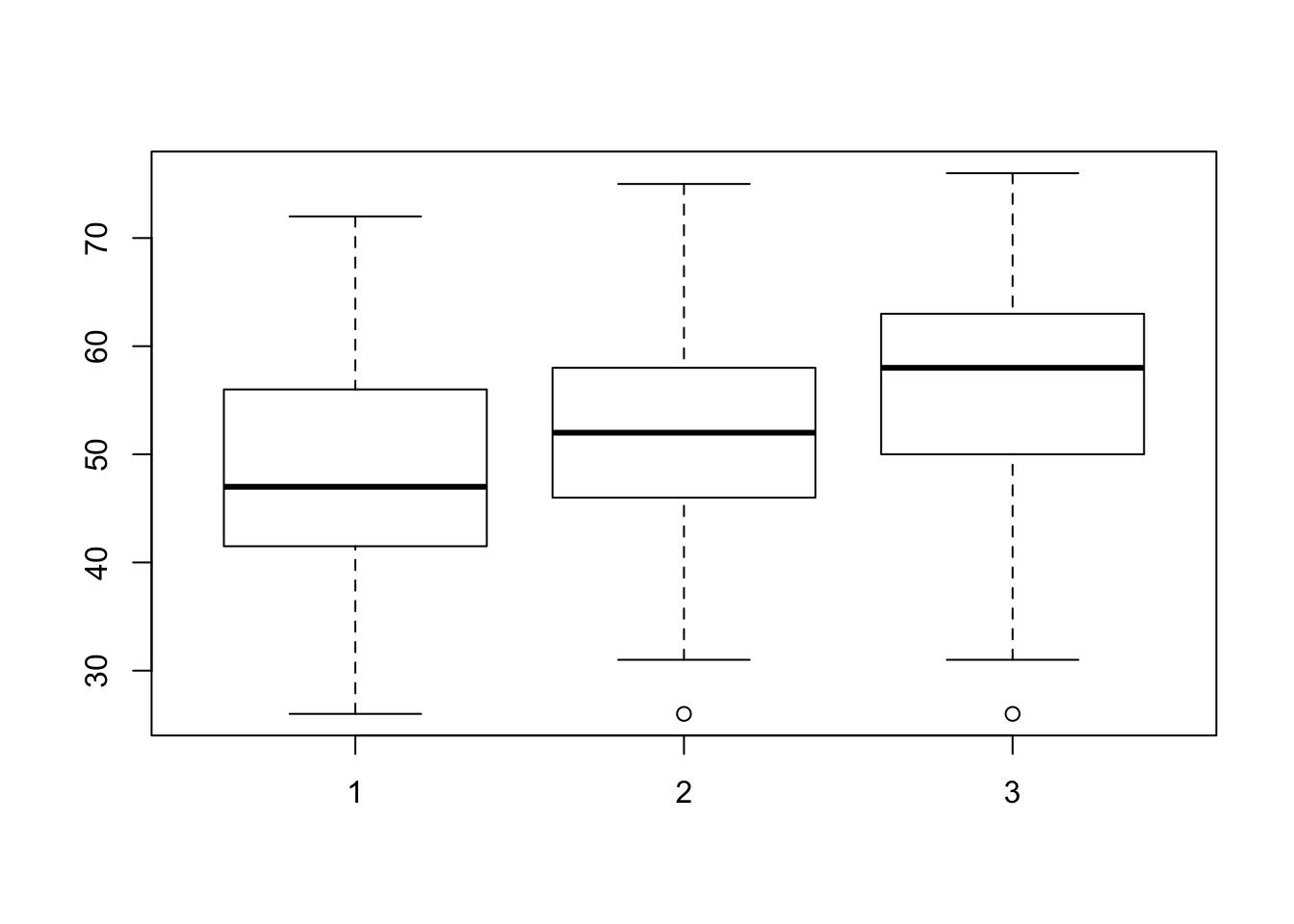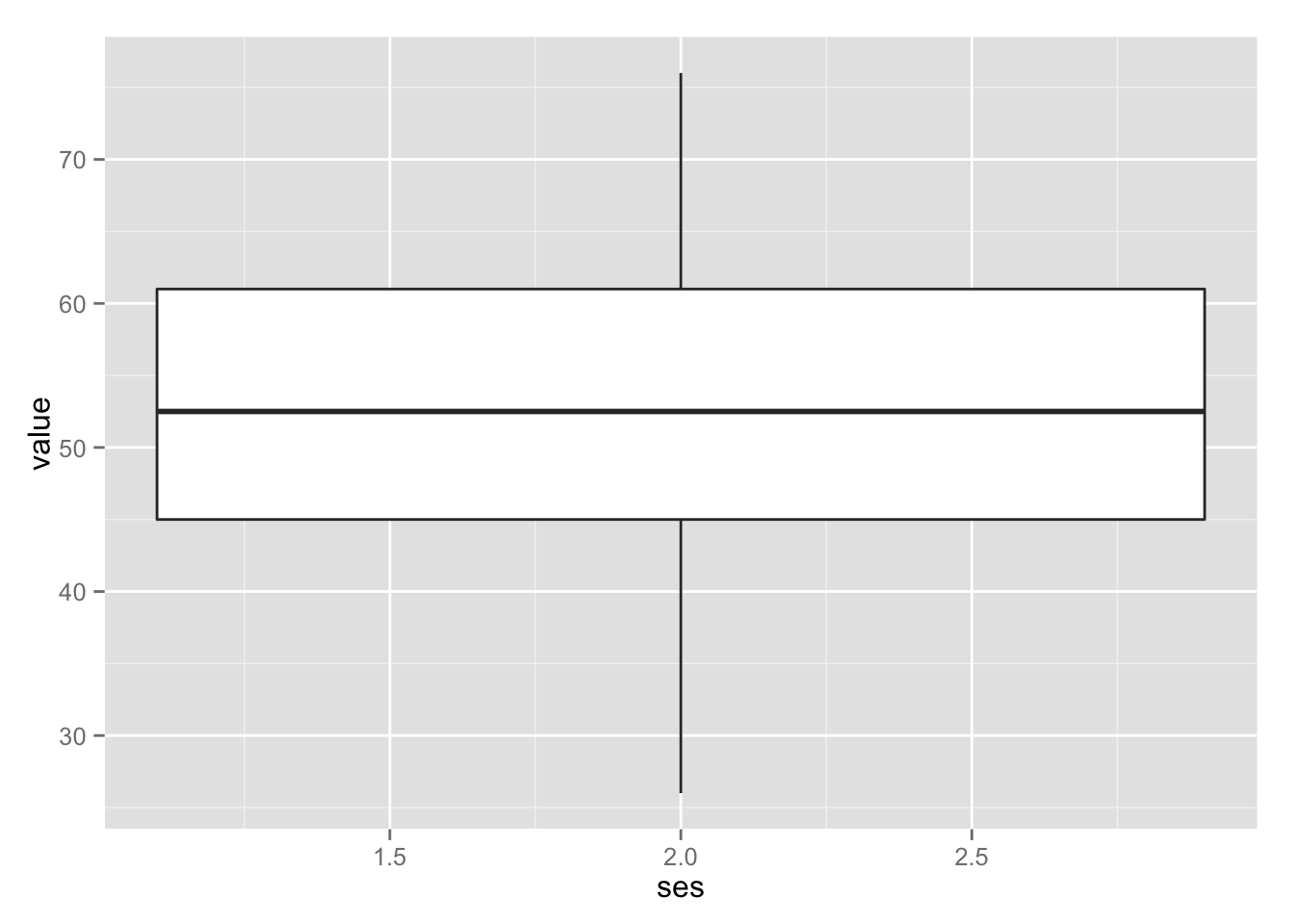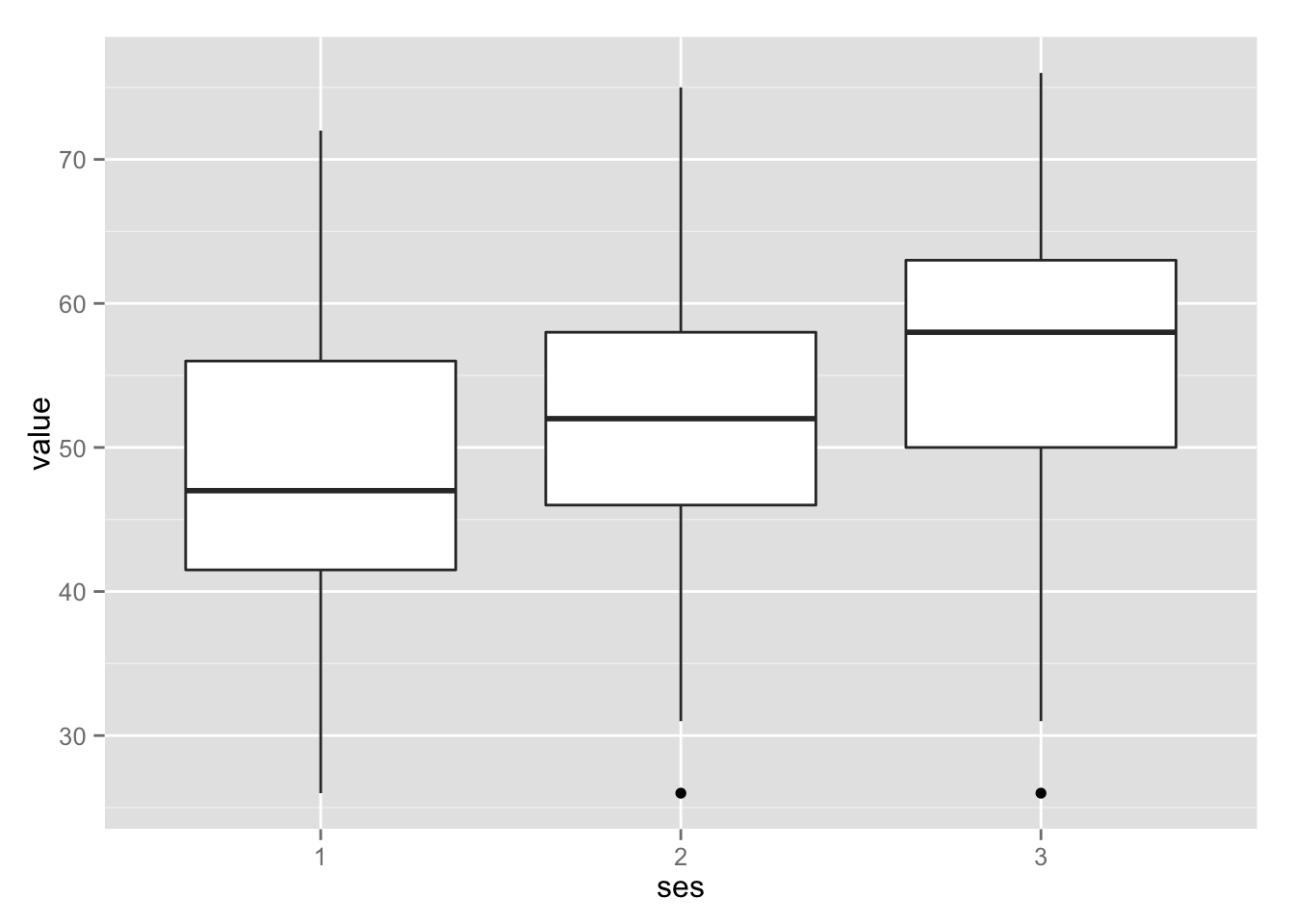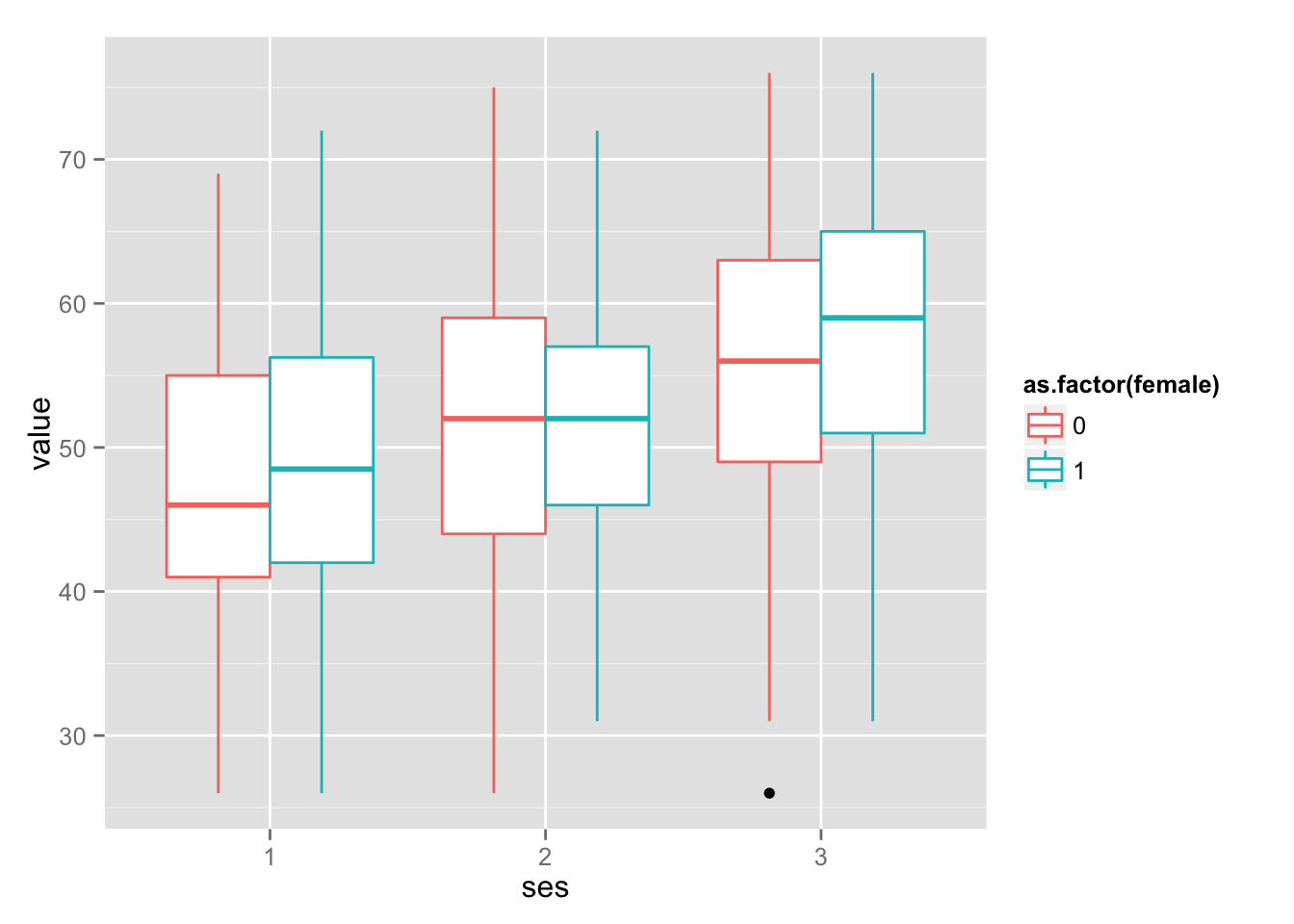## In base R

At this point, I’m going to move ahead to plots, before coming back to other ways to manipulate data frames. The reason is simple: plotting my data is usually my first pass at seeing what’s going on. I usually manipulate my data frames for the purpose of plotting it in a particular way, and seeing the data first gives me better ideas of how to manipulate the data frame in order to plot it more informatively.

For the `hs_sorted` data, one question we might ask is how socioeconomic status affects test scores. A boxplot will start to answer this question informatively. In base R, we use the function `boxplot()` to do this. In this function, we see a new character `~`. We read this as “by”. The function below can be read as “make a plot of how the test score value varies by socioeconomic status. Use `hs_sorted` as the data”.

``boxplot(value ~ ses, data = hs_sorted)``This is a nice first pass at the data. We learn that test scores go up for higher SES scores. Other plot commands in base R include:

Function Does
`boxplot(y ~ x, data = data_frame)` boxplot
`plot(y ~ x, data = data_frame)` scatter plot
`hist(data_frame\$column)` histogram
`density(data_frame\$column)` density plot

For a detailed look at graphics in base R, check out Joe’s R Study Group.

## Making it nice with `ggplot`

I find R’s base plots actually much harder to deal with than `ggplot` (which stands for “graphics of grammar plot”), since base plots are hard to control - so my first step in looking at data is actually to plot it using `ggplot`. Today, I’ll introduce `ggplot`, and tomorrow we’ll go into more detail.

`ggplot` is a package that give you a lot of freedom over your plots. Its defaults are set to make beautiful plots, which - let’s face it - makes it easier to convince people that your data is the real deal. My go-to for help with `ggplot` is Winston Chang’s Cookbook for R. Again, Joe’s R Study Group is also a great tutorial, and Hadley Wickham’s (the author of ggplot) paper on ggplot provides some more in-depth info on the theory behind `ggplot` as a data visualization tool. I especially recommend reading Section 7 (just one page long) Here’s a teaser:

How can we build on top of the grammar to help data analysts build compelling, revealing graphics? What would a poetry of graphics look like?

Who doesn’t want their graphics to be compelling and revealing - poetic in their informativeness!? I’m sold. `ggplot` works a little differently than plotting in base R, because it’s not just a single line of code.

With `ggplot`, you’re creating a plot out of layers. The main types of layers that you’ll add are:

• Data and Aesthetics. The data is obviously an important part of the plot. Along with the dataset, we also need to know how to map the data onto visual aesthetics. Aesthetics assigns a variable to x and y, as well as designates other aesthetic ways to represent the data (variables by color or shape, for example). In `ggplot`, this is shortened to `aes()`.

• Geometrics, or `geom` objects. This tells `ggplot` what kind of geometrics to put on the graph. Is it points, as in a scatter plot (`geom_point()`)? Is it a box plot (`geom_box)

• Statistics, or `stat` layers. These layers perform summary statistics on the data. And example of a `stat` layer is adding a smoothed line to a scatterplot, with `stat_smooth()`.

• Scales and themes are layers that you’ll add to fine-tune the plot - give it the color scheme that you want, or the background shade that you want.

### Let’s try it out!

We’ll start with the `ggplot` equivalent of the boxplot that we made above in base graphics. First we need to install and load `ggplot2`.

``````install.packages("ggplot2")
library(ggplot2)``````
``````##
## Attaching package: 'ggplot2'
##
## The following object is masked _by_ '.GlobalEnv':
##
##     movies``````
``head(hs_sorted)``
``````##     id female race ses schtyp prog variable value
## 99   1      1    1   1      1    3     read    34
## 299  1      1    1   1      1    3    write    44
## 499  1      1    1   1      1    3     math    40
## 699  1      1    1   1      1    3  science    39
## 899  1      1    1   1      1    3    socst    41
## 139  2      1    1   2      1    3     read    39``````

The basic way to create a plot is to put the data and aesthetics in the `ggplot()` function, and then add the layers of geom and stat that you want. The first line calls the data frame as the first argument, and then the list of aesthetic mappings as the second argument.

`ggplot(data_frame, aes(x = x_var, y = y_var))`

After adding the data, we can make that data materialize with whatever geometric layer we want. We achieve this by adding a `+` to the end of the first line, so that the function knows its not done yet. Most style guides will suggest adding each layer on its own line, to keep the code maximally readable.

``````ggplot(hs_sorted, aes(x = ses, y = value)) +
geom_boxplot()``````Something’s wrong here … Why is `ggplot` only giving us one overall boxplot instead of a separate boxplot for each ses? The answer has to do with how the data is coded. If we take a look at the `summary` of `hs_sorted`, we can see that R thinks that `ses` is a measurement on a scale. It tells us that 2.055 is the mean socioeconomic status of this dataset. But this is silly: we know that students are given a discrete score of either 1, 2, or 3. In other words, even though `ses` is represented as a numeric variable, it’s actually a factor variable. It increases in discrete steps.

``summary(hs_sorted)``
``````##        id             female           race           ses
##  Min.   :  1.00   Min.   :0.000   Min.   :1.00   Min.   :1.000
##  1st Qu.: 50.75   1st Qu.:0.000   1st Qu.:3.00   1st Qu.:2.000
##  Median :100.50   Median :1.000   Median :4.00   Median :2.000
##  Mean   :100.50   Mean   :0.545   Mean   :3.43   Mean   :2.055
##  3rd Qu.:150.25   3rd Qu.:1.000   3rd Qu.:4.00   3rd Qu.:3.000
##  Max.   :200.00   Max.   :1.000   Max.   :4.00   Max.   :3.000
##      schtyp          prog          variable       value
##  Min.   :1.00   Min.   :1.000   read   :200   Min.   :26.00
##  1st Qu.:1.00   1st Qu.:2.000   write  :200   1st Qu.:45.00
##  Median :1.00   Median :2.000   math   :200   Median :52.50
##  Mean   :1.16   Mean   :2.025   science:200   Mean   :52.38
##  3rd Qu.:1.00   3rd Qu.:2.250   socst  :200   3rd Qu.:61.00
##  Max.   :2.00   Max.   :3.000                 Max.   :76.00``````

Factors are actually a litte complicated. Underlyingly, they are numeric values that are represented with character strings. This mostly has implications for statistical analysis, which we won’t be getting into in this mini course. But it’s good to know that when you have e.g, gender represented as a factor (which is R’s default representation for character variables), it is underlying a series of 0 and 1.

For the purpose of plotting the data in an informative way, all we need to do is realize that the `ses` variable looks linear to `ggplot`, but that we intend it to be a factor. Easy! We can coerce variables into other data types by using the following:

Function Does
`as.factor()` coerce to factor
`as.character()` coerce to character string
`as.numeric()` coerce to numbers

For this data, we need to coerce `ses` to a factor. We can do this either outside of the `ggplot` function or within it. The following are equivalent:

``````## change the data frame and then plot it (good for if you want to continue working with ses as a factor)

hs_sorted\$ses <- as.factor(hs_sorted\$ses)
ggplot(hs_sorted, aes(x = ses, y = value)) +
geom_boxplot()

## change the variable just when you plot it
ggplot(hs_sorted, aes(x = as.factor(ses), y = value)) +
geom_boxplot()``````

Both of these options produce the graph we’re looking for:### More ways to visualize with `ggplot`

Okay great, so we made the same graph as before, and it looks a little bit nicer. But we’re just getting started! One of the biggest benefits of using `ggplot` as a first pass at your data is the flexible aesthetics. With `ggplot`, we can assign color or shape based on a variable - which is something we can’t do in base R1 that takes an extra step to do in base R.

Let’s see whether gender has an additional effect on test scores. Note that the gender variable gives us the same problem as the socioeconomic status variable, so we have to also coerce it to a factor first.

``````ggplot(hs_sorted, aes(x = ses, y = value, color = as.factor(female))) +
geom_boxplot()``````Beautiful! What else might be informative? Maybe there’s a difference in subject score by socioeconomic status, or by gender? Note that here I’m also changing the `color` aesthetic mapping to a `fill`, because I want the boxes to be filled with color instead of just changing the color of the lines.

``````ggplot(hs_sorted, aes(x = variable, y = value, fill = ses)) +
geom_boxplot() ``````